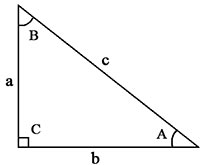# Pythagoras Calculator

Before using the Pythagoras Calculator, it's important to note that all angles in this case are measured in degrees, not radians.

A right-angled triangle has three angles: one 90-degree angle and two acute angles, which we'll call Angle A and Angle B. The sum of the two acute angles is always 90 degrees, giving a total of 180 degrees.

To use the calculator, input the value of one known dimension of the triangle: the length of the adjacent side, the opposite side, or the hypotenuse. The adjacent side is the side that forms the angle with the hypotenuse, while the opposite side is opposite to the given angle.

The Pythagorean theorem states that the sum of the squares of the adjacent and opposite sides is equal to the square of the hypotenuse. The formula is c2 = a2 + b2, where c is the hypotenuse and a and b are the adjacent and opposite sides, respectively.

Pythagoras Calculator°

°

°

sq. units

You may set the number of decimal places in the online calculator. By default there are only two decimal places.

Changing the value of one dimension will affect the values of the other dimensions. For example, changing the value of Angle A will affect the value of Angle B, the length of the adjacent side, the length of the hypotenuse, and the area of the triangle. Similarly, changing the value of Angle B will affect the value of Angle A, the length of the opposite side, the length of the hypotenuse, and the area of the triangle.

If you change the length of the opposite side, it will affect the values of both Angle A and Angle B, as well as the length of the hypotenuse and the area of the triangle. Changing the length of the adjacent side will also affect the values of Angle A and Angle B, the length of the hypotenuse, and the area of the triangle. Finally, if you change the length of the hypotenuse, it will affect the length of the opposite and adjacent sides, as well as the area of the triangle.